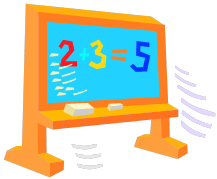page contents

Math Curriculum and Resources

For our math program, we will be using the Go Math! series.

We will discuss the following units throughout the school year:

Chapter 1: Addition and Subtraction within 1,000

Chapter 2: Represent and Interpret Data

Chapter 3: Understand Multiplication

Chapter 4: Multiplication Facts and Strategies

Chapter 5: Use Multiplication Facts

Chapter 6: Understand Division

Chapter 7: Division Facts and Strategies

Chapter 8: Understanding Fractions

Chapter 9: Compare Fractions

Chapter 10: Time, Length, Liquid Volume, and Mass

Chapter 11: Perimeter and Area

Chapter 12: Two-Dimensional Shapes